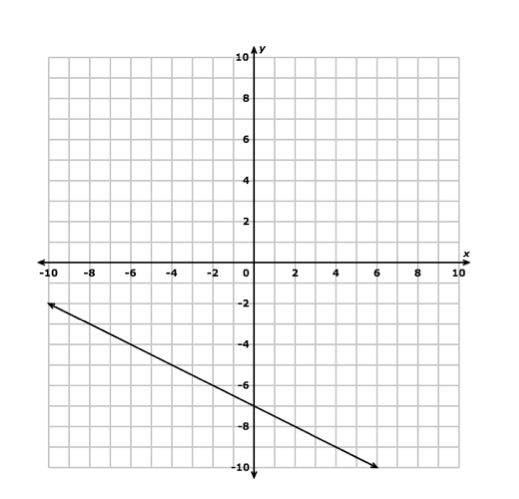+
Writing an equation in Slope-Intercept form from a Graph
Chapter 5 Algebra 1 Linear Equations

# Writing an equation in Slope-Intercept form from a Graph

##### Rating:
(0)
Author: Laura Martin
Tutorial

## Writing an Equation in Slope-Intercept Form from a GRAPH

This tutorial will demonstrate and explain how to use a graph of a linear equation to write the equation in slope-intercept form.

## The equation for the second graph!

We found the slope to be -1/2

The y-intercept is -7

The equation in slope-intercept form is :

y = -1/2x - 7Find 3 ordered pairs that are solutions for this equation.

1.

2.

3.

Rating Header